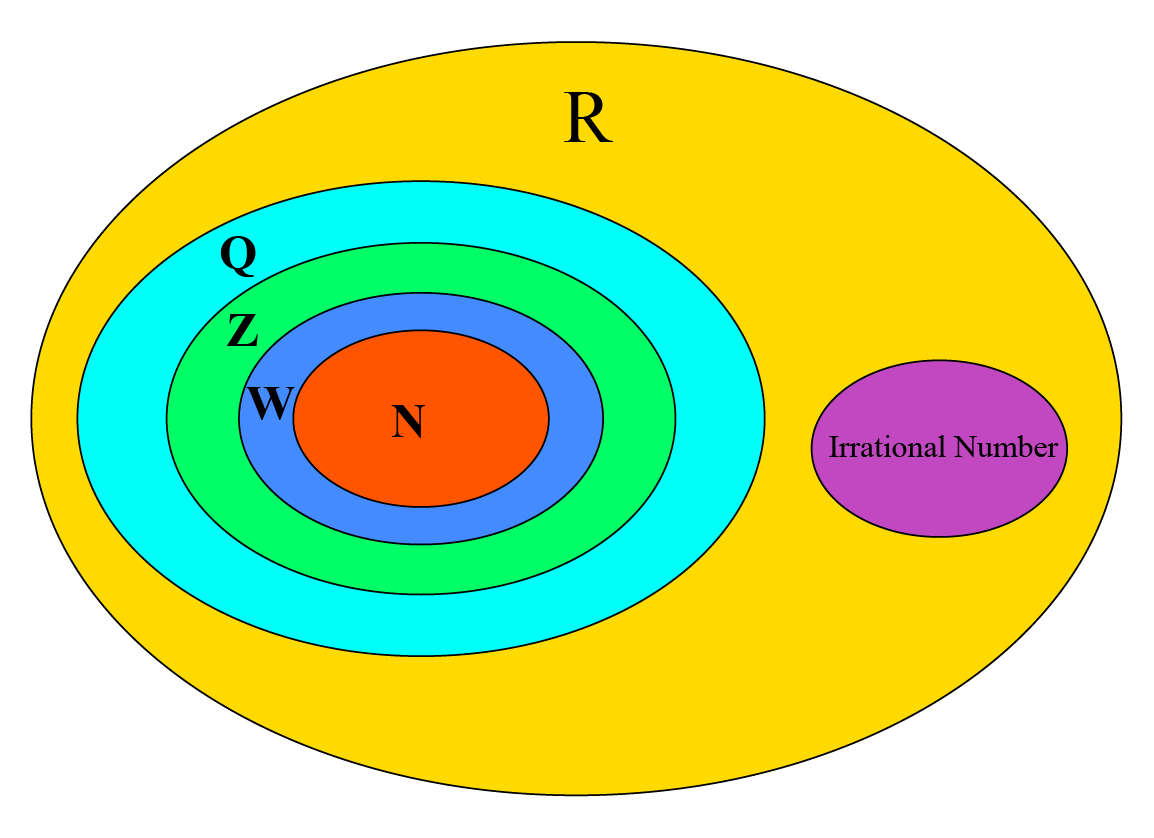# Real NumbersReal numbers? Sounds strange? How can numbers be real or not real? Let us understand the magic of numbers:

Real number (R) is a big bucket in which you pour in:

1. Natural Numbers (N)

2. Whole Numbers (W)

3. Integers (Z)

4. Rational Numbers (Q)

5. Irrational NumberI am sure, many more questions must be bothering you, like what are these  different numbers?

•  We know, Natural Numbers are the positive numbers starting from 1, i.e. 1,2,3…..

• 0 plus all the natural numbers makes the set of Whole Numbers, i.e. 0, 1,2,3…..

• All the negatives of the natural numbers and the whole numbers make up the Integers, i.e. ….-3, -2, -1, 0, 1,2,3…..

All these numbers can be represented on the Number Line. But does it complete the number line?

Every point on the number line represents a Real NumberWhat are these fractional numbers? What is  𝝅?

Any number which can be represented in the form of p/q , where q ≠ 0, is known as rational numbers.

Like 3/4 , 25 = 25/1

So we can say, every integer is a Rational Number.

𝝅 = 3.1459…….

So we can understand, 𝝅 is a number, which cannot be expressed in simple fractions.

What about the numbers which cannot be expressed in the form of p/q?

These numbers are known as irrational numbers, like, 𝝅 , square root of a non – perfect  square (√3)

Does this mean, every number is a Real Number?

No, there are numbers, which are not real.

The non - real numbers are;

• Imaginary numbers, like the square root of -1 (√-1)

• Infinity